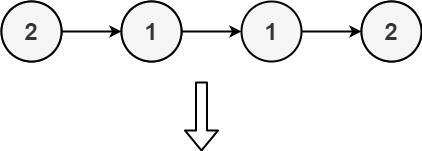## 1836. Remove Duplicates From an Unsorted Linked List

Given the `head` of a linked list, find all the values that appear more than once in the list and delete the nodes that have any of those values.

Return the linked list after the deletions.

Example 1:```Input: head = [1,2,3,2]
Output: [1,3]
Explanation: 2 appears twice in the linked list, so all 2's should be deleted. After deleting all 2's, we are left with [1,3].
```

Example 2:```Input: head = [2,1,1,2]
Output: []
Explanation: 2 and 1 both appear twice. All the elements should be deleted.
```

Example 3:```Input: head = [3,2,2,1,3,2,4]
Output: [1,4]
Explanation: 3 appears twice and 2 appears three times. After deleting all 3's and 2's, we are left with [1,4].
```

Constraints:

• The number of nodes in the list is in the range `[1, 105]`
• `1 <= Node.val <= 105`

## Rust Solution

``````struct Solution;
use rustgym_util::*;
use std::collections::HashMap;

impl Solution {
let mut nodes: Vec<Box<ListNode>> = vec![];
let mut hm: HashMap<i32, usize> = HashMap::new();
while let Some(mut node) = p {
p = node.next.take();
let val = node.val;
*hm.entry(val).or_default() += 1;
nodes.push(node);
}
let mut res = None;
while let Some(mut node) = nodes.pop() {
if hm[&node.val] == 1 {
node.next = res;
res = Some(node);
}
}
res
}
}

#[test]
fn test() {
let head = list![1, 2, 3, 2];
let res = list![1, 3];